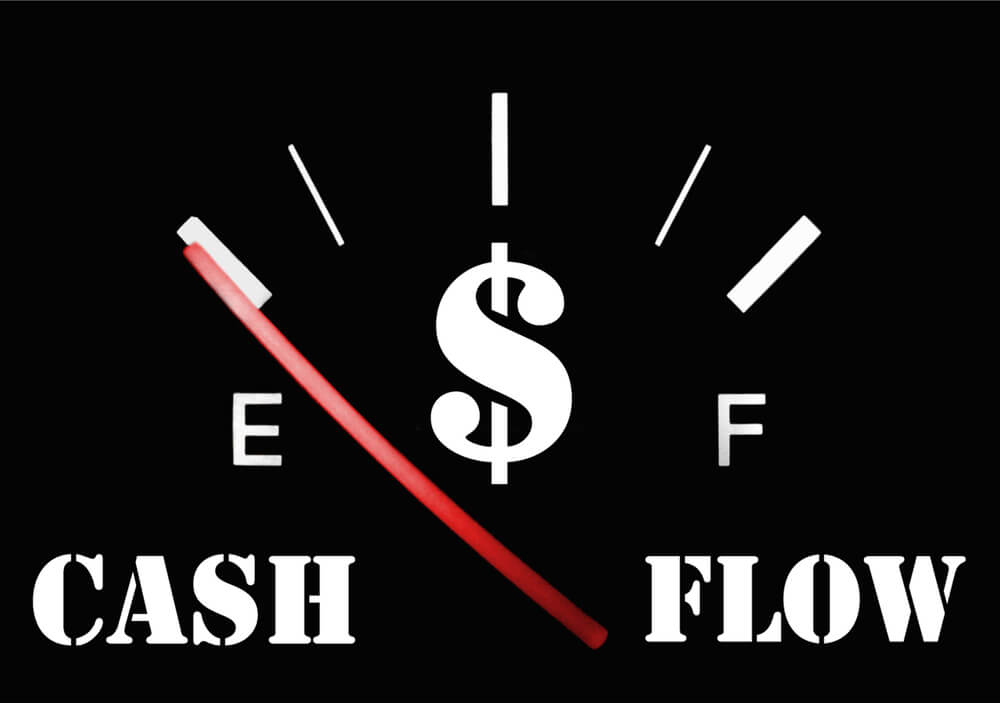# What is the Difference Between Discounted and Undiscounted Cash Flows?A fundamental concept in investments is the time value of money — the idea that there is a greater benefit to receiving a sum of money now, rather than an identical sum later.

It also takes into account the reduction in the real value of funds from the effects of inflation.

There is, however, a key difference between discounted and undiscounted cash flows: discounted cash flows have been adjusted to incorporate the time value of money, unlike undiscounted cash flows which aren’t adjusted to include the time value of money.

When these two methods are used to evaluate an investment, the outcomes will be significantly different, so it’s essential to know how to tell them apart.

## What is Discounted Cash Flow?

In finance, DCF stands for discounted cash flow, which is an analysis method of valuing a project, asset, company, or security using the concepts of the time value of money.

It started in the industry as early as the 1700s or 1800s and was then widely discussed in the 1960s in financial economics until it became widely accepted by the U.S. courts in the ‘80s and ‘90s.

Discounted cash flows are adjusted to incorporate the time value of money — they are discounted using a discount rate to get the present value estimate, which is used to evaluate the potential for investment.

Discounted cash flows can be calculated using this formula:

Discounted cash flows= CF 1/ (1+r) 1 + CF 2/ (1+r) 2 +… CF n (1+r) n

Where: CF = Cash flow and r = Discount rate.

By using the formula above, discounted cash flows can easily be calculated if there’s limited cash flow.

However, this isn’t a convenient formula to use if there are many cash flows.

Discounted cash flows are most suitable to use for evaluating investment decisions by comparing the discounted cash inflows and cash outflows. NPV, known as Net Present Value, is a technique for investment appraisal that uses discounted cash flows to find out if a project is financially feasible.

### Example of Discounted Cash Flow

Before a company makes the decision to invest in a certain project or commit to buying new equipment, they will analyze their weighted average cost of capital or WACC as a discount rate when evaluating the DCF.

The WACC includes the average rate of return that shareholders in the firm are expecting for the given year.

So if you’re looking to invest in a project and your company has a WACC of 5%, then 5% is your discount rate.

For example, let’s say that the initial investment is \$11 million and the project will take 5 years to complete. This is what the breakdown would look like:

#### Discounted Cash Flow

Summing up all the discounted cash flows, we would get a total value of \$13,306,749, and when we subtract the initial investment of \$11 million, we get an NPV of \$2,306,749.

Because of this positive number, we can say that the investment we put in today will be worth it since the project will generate discounted cash flows above the initial cost.

However, if the project had cost \$14 million, the NPV would’ve been -\$693,251, which means that the cost of investment wouldn’t be worth it.

## Disadvantages of Discounted Cash Flow

The biggest flaw of DCF is that it requires making a lot of assumptions — an investor would have to correctly estimate the future cash flows from a project or investment. Because of this, future cash flows would need to rely on

various factors, such as the status of the economy, the market demand, unforeseen obstacles, and more.
Making estimates for the cash flow is a risky move and could lead to choosing investments that may not pay off in the future, and thus hurting profits.

Even by estimating cash flows too low can have dire consequences that can end in missed opportunities.

Choosing a discount rate also relies on assumptions and will need to be estimated correctly for the model to be worthwhile.

## Undiscounted Cash Flows

Undiscounted cash flows don’t incorporate the time value of money, and is the opposite of discounted cash flows — they solely consider the normal value of cash flows when it comes to making investment decisions.

Because undiscounted cash flows don’t consider the reduction in the value of money over time, it isn’t used to assist accurate investment decisions.

While undiscounted cash flows may seem to generate a positive NPV at first, they won’t be able to generate the same amount after a few years down the road.

This is because the effect of time and money isn’t applied, therefore, NPV can be heavily overstated.

Furthermore, undiscounted future cash flows are cash flows that are expected to be incurred or generated by a project which hasn’t been reduced to their present value.

This may happen when interest rates are near zero, or the expected cash flows cover such a short period of time that the use of a discount wouldn’t result in a different outcome.

## Discounted vs Undiscounted Cash Flows

Here are the main differences between discounted and undiscounted cash flows: